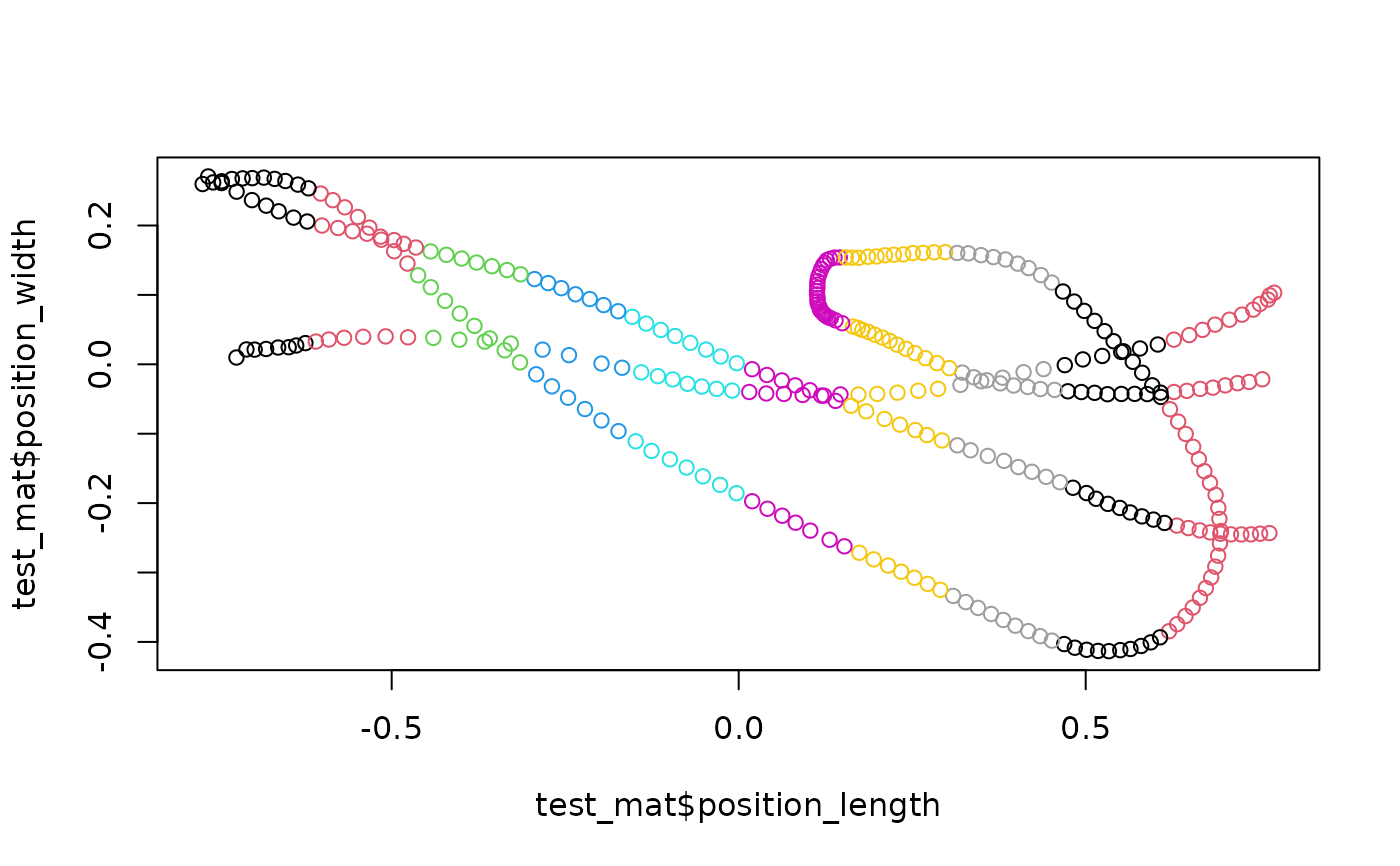Chop data into X sections (of equal size) along a specified axis

## Usage

``section_tunnel_by(obj_name, axis = "position_length", number_of_sections = 20)``

## Arguments

obj_name

The input viewr object; a tibble or data.frame with attribute `pathviewr_steps` that includes `"viewr"`

axis

Chosen axis, must match name of column exactly

number_of_sections

Total number of sections

## Value

A new column added to the input data object called `section_id`, which is an ordered factor that indicates grouping.

## Details

The idea is to bin the data along a specified axis, generally `position_length`.

Vikram B. Baliga

## Examples

``````## Load data and run section_tunnel_by()
test_mat <-
package = 'pathviewr'),
subject_name = "birdie_wooster") %>%
redefine_tunnel_center(length_method = "middle",
height_method = "user-defined",
height_zero = 1.44) %>%
select_x_percent(desired_percent = 50) %>%
separate_trajectories(max_frame_gap = 1) %>%
get_full_trajectories(span = 0.95) %>%
section_tunnel_by(number_of_sections = 10)

## Plot; color by section ID
plot(test_mat\$position_length,
test_mat\$position_width,
asp = 1, col = as.factor(test_mat\$section_id))``````